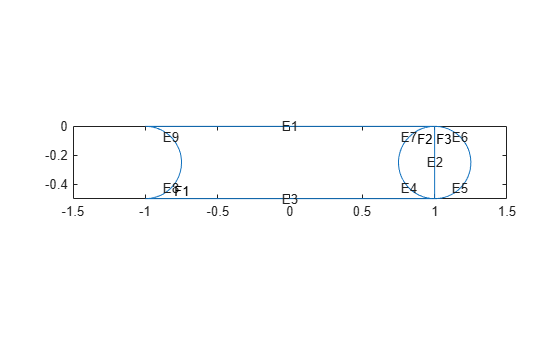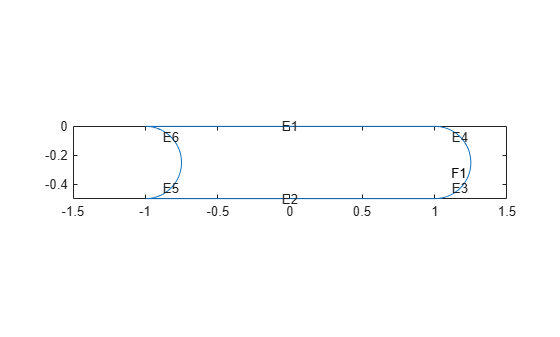Documentation

2-D Geometry Creation at Command Line

Three Elements of Geometry

To describe your geometry through Constructive Solid Geometry (CSG) modeling, use three data structures.

1. A matrix whose columns describe the basic shapes. When you export geometry from the PDE Modeler app, this matrix has the default name gd (geometry description). See Create Basic Shapes.

2. A matrix whose columns contain names for the basic shapes. Pad the columns with zeros or 32 (blanks) so that every column has the same length. See Create Names for the Basic Shapes.

3. A set of characters describing the unions, intersections, and set differences of the basic shapes that make the geometry. See Set Formula.

Create Basic Shapes

To create basic shapes at the command line, create a matrix whose columns each describe a basic shape. If necessary, add extra zeros to some columns so that all columns have the same length. Write each column using the following encoding.

Circle

RowValue
11 (indicates a circle)
2x-coordinate of circle center
3y-coordinate of circle center

Polygon

RowValue
12 (indicates a polygon)
2Number of line segments n
3 through 3+n-1x-coordinate of edge starting points
3+n through 2*n+2y-coordinate of edge starting points

Note

Your polygon cannot contain any self-intersections. To check whether your polygon satisfies this restriction, use the csgchk function.

Rectangle

RowValue
13 (indicates a rectangle)
24 (number of line segments)
3 through 6x-coordinate of edge starting points
7 through 10y-coordinate of edge starting points

The encoding of a rectangle is the same as that of a polygon, except that the first row is 3 instead of 2.

Ellipse

RowValue
14 (indicates an ellipse)
2x-coordinate of ellipse center
3y-coordinate of ellipse center
4First semiaxis length (strictly positive)
5Second semiaxis length (strictly positive)
6Angle in radians from x axis to first semiaxis

For example, specify a matrix that has a rectangle with a circular end cap and another circular excision. First, create a rectangle and two adjoining circles.

rect1 = [3
4
-1
1
1
-1
0
0
-0.5
-0.5];
C1 = [1
1
-0.25
0.25];
C2 = [1
-1
-0.25
0.25];

Append extra zeros to the circles so they have the same number of rows as the rectangle.

C1 = [C1;zeros(length(rect1) - length(C1),1)];
C2 = [C2;zeros(length(rect1) - length(C2),1)];

Combine the shapes into one matrix.

gd = [rect1,C1,C2];

Create Names for the Basic Shapes

In order to create a formula describing the unions and intersections of basic shapes, you need a name for each basic shape. Give the names as a matrix whose columns contain the names of the corresponding columns in the basic shape matrix. Pad the columns with 0 or 32 if necessary so that each has the same length.

One easy way to create the names is by specifying a character array whose rows contain the names, and then taking the transpose. Use the char function to create the array. char pads the rows as needed so all have the same length. Continuing the example, give names for the three shapes.

ns = char('rect1','C1','C2');
ns = ns';

Set Formula

Obtain the final geometry by writing a set of characters that describes the unions and intersections of basic shapes. Use + for union, * for intersection, - for set difference, and parentheses for grouping. + and * have the same grouping precedence. - has higher grouping precedence.

Continuing the example, specify the union of the rectangle and C1, and subtract C2.

sf = '(rect1+C1)-C2';

Create Geometry and Remove Face Boundaries

After you have created the basic shapes, given them names, and specified a set formula, create the geometry using decsg. Often, you also remove some or all of the resulting face boundaries. Completing the example, combine the basic shapes using the set formula.

[dl,bt] = decsg(gd,sf,ns);

View the geometry with and without boundary removal.

pdegplot(dl,'EdgeLabels','on','FaceLabels','on')
xlim([-1.5,1.5])
axis equalRemove the face boundaries.

[dl2,bt2] = csgdel(dl,bt); % removes face boundaries
figure
pdegplot(dl2,'EdgeLabels','on','FaceLabels','on')
xlim([-1.5,1.5])
axis equalDecomposed Geometry Data Structure

A decomposed geometry matrix has the following encoding. Each column of the matrix corresponds to one boundary segment. Any 0 entry means no encoding is necessary for this row. So, for example, if only line segments appear in the matrix, then the matrix has 7 rows. But if there is also a circular segment, then the matrix has 10 rows. The extra three rows of the line columns are filled with 0.

RowCircleLineEllipse
1124
2Starting x coordinateStarting x coordinateStarting x coordinate
3Ending x coordinateEnding x coordinateEnding x coordinate
4Starting y coordinateStarting y coordinateStarting y coordinate
5Ending y coordinateEnding y coordinateEnding y coordinate
6Region label to left of segment, with direction induced by start and end points (0 is exterior label)Region label to left of segment, with direction induced by start and end points (0 is exterior label)Region label to left of segment, with direction induced by start and end points (0 is exterior label)
7Region label to right of segment, with direction induced by start and end points (0 is exterior label)Region label to right of segment, with direction induced by start and end points (0 is exterior label)Region label to right of segment, with direction induced by start and end points (0 is exterior label)
8x coordinate of circle center0x coordinate of ellipse center
9y coordinate of circle center0y coordinate of ellipse center
10Radius0Length of first semiaxis
1100Length of second semiaxis
1200Angle in radians between x axis and first semiaxis
Partial Differential Equation Toolbox Documentation평가판 신청